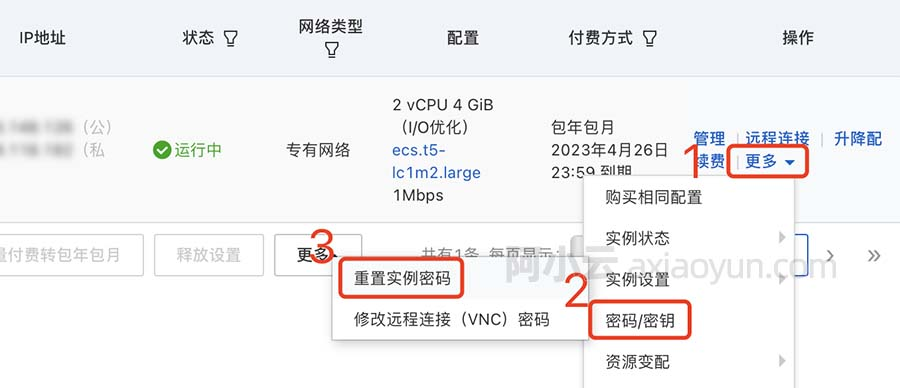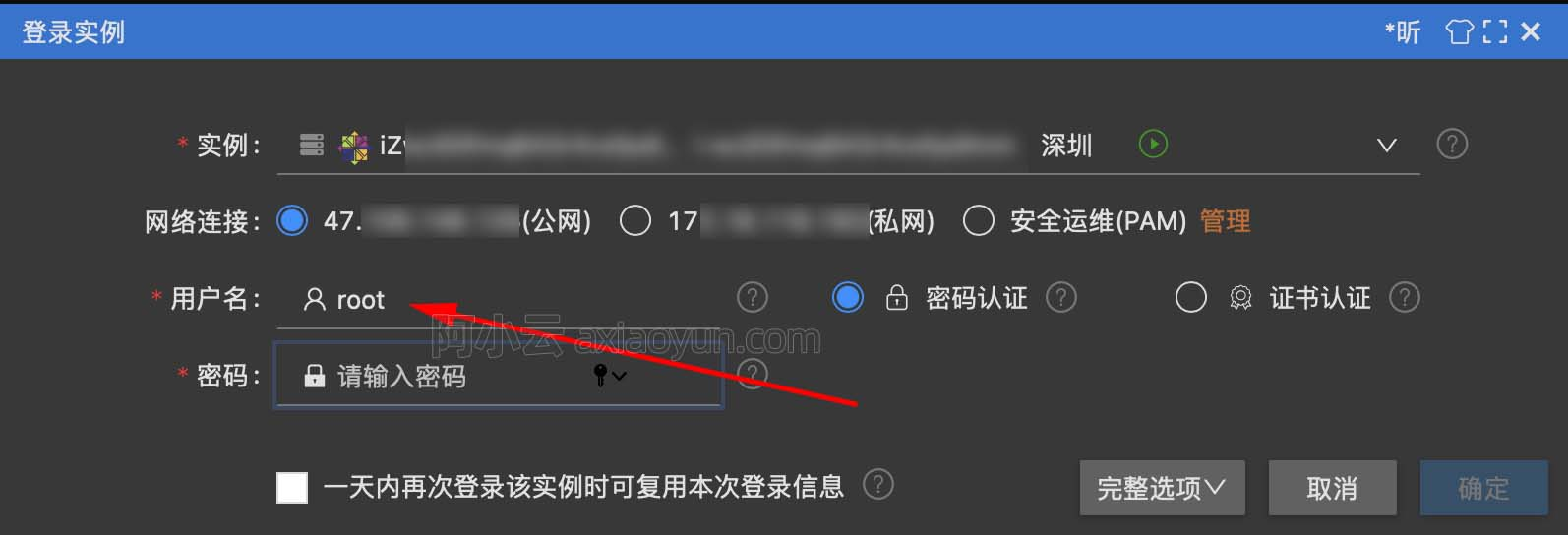传说中的贝叶斯统计到底有什么来头？

+关注继续查看1. 频率统计2. 频率统计的内在缺陷

1. p-values 对固定大小的样本进行检测。如果两个人对相同的数据工作，并有不同的制动意向，他们可能会得到两种不同的p- values

2. 置信区间（CI）和p-value一样，在很大程度上取决于样本的大小。因为无论多少人如何执行相同的数据测试，其结果应该是一致的。

3. 置信区间（CI）不是概率分布，因此它们不提供最可能的值以及其参数。

3.贝叶斯统计

“贝叶斯统计是将概率运用到统计问题中的数学过程。它提供给人们工具来更新数据中的证据。”要更好的理解这个问题，我们需要对于一些概念要有所认识。此外，也需要有一定的前提：

3.1条件概率P（A）= 1/2，由于每俩天都会下一次雨。

P（B）为1/4，因为詹姆斯每四次比赛只赢一次。

P（A | B）= 1，因为每次下雨James都赢。

3.2 贝叶斯定理4.贝叶斯推理

4个硬币头朝上的概率即给定硬币（θ）的公平性，即P(D|θ)

P(θ|D)=(P(D|θ) X P(θ))/P(D)

P(D|θ) 是考虑到我们给定分布θ时，我们结果的可能性。如果我们知道硬币是公平的，这就是观测到的头朝上的概率。

P(D)就是证据，这是因为通过在θ的所有可能的值，是θ的那些特定值加权求和（或积分）确定的数据的概率。

P(θ|D) 是观察，即头在上数目之后我们的参数。

4.1 伯努利近似函数

P(y=1|θ)=[如果硬币是公平的θ= 0.5，观察到头的概率（Y = 1）为0.5]

P(y=0|θ)=[如果硬币是公平的θ= 0.5，观察到尾部的概率（Y = 0）为0.5]

P（Y |θ）=y={0,1},θ=(0,1)4.2 前置信度分布

Beta分布的概率密度函数的形式为：α和 β被称为形状决定密度函数的参数。这里α类似于试验中出现头的数量，β对应于实验中尾的数量。下图将帮助您想象不同值中 α和 β的测试分布> library(stats)
> par(mfrow=c(3,2))
> x=seq(0,1,by=o.1)
> alpha=c(0,2,10,20,50,500)
> beta=c(0,2,8,11,27,232)
> for(i in 1:length(alpha)){
y<-dbeta(x,shape1=alpha[i],shape2=beta[i])
plot(x,y,type="l")
}4.3 后置信度分布prior = P(θ|α,β)=P(θ|13.8,9.2)

Posterior = P(θ|z+α,N-z+β)=P(θ|93.8,29.2)> library(stats)
> x=seq(0,1,by=0.1)
> alpha=c(13.8,93.8)
> beta=c(9.2,29.2)
> for(i in 1:length(alpha)){
y<-dbeta(x,shape1=alpha[i],shape2=beta[i])
plot(x,y,type="l",xlab = "theta",ylab = "density")

}

5. 测试意义——频率论VS贝叶斯

5.1 p值

5.2 置信区间

5.3 贝叶斯因子θ的各种值代表贝叶斯统计调整可信度（概率）。可以很容易地看出，概率分布已经转向M2具有更高的值M1，即M2更可能发生。5.4 高密度间隔（HDI）

HDI由后验分布观察新数据形成，由于HDI是一个概率，95％的HDI给出95％的最可信的值。它也保证了95％的值将处于不同的CI区间。10098 026797 06767 014503 011640 0windows server 2008阿里云ECS服务器安全设置

9161 013895 07365 054769 04511 0
3598

840

+ 订阅

《2021云上架构与运维峰会演讲合集》

《零基础CSS入门教程》

《零基础HTML入门教程》